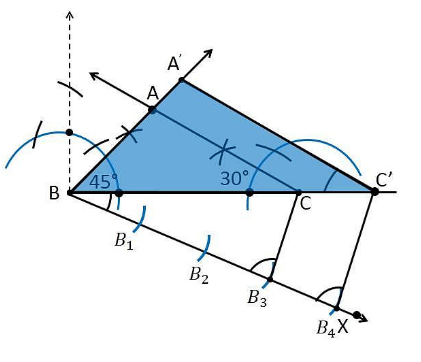# Draw a triangle ABC with side BC = 7 cm, ∠ B = 45°, ∠ A = 105°. Then, construct a triangle whose sides are 4/3 times the corresponding sides of ∆ ABC. Give the justification of the construction

Let us first find the measure of ∠C

∠B = 45°, ∠A = 105°

We know that,

The Sum of all interior angles in a triangle is 180°.

∠A+∠B +∠C = 180°

105°+45°+∠C = 180°

∠C = 180° − 150°

∠C = 30°

To construct a triangle from given conditions

Construction Procedure:

The required triangle can be drawn as follows.

1. Draw a ΔABC with side measures of base BC = 7 cm, ∠B = 45°, and ∠C = 30°.

2. Draw a ray BX makes an acute angle with BC on the opposite side of vertex A.

3. Locate 4 points (as 4 is greater in 4 and 3), such as B1, B2, B3, B4, on the ray BX.

4. Join the points B3C.

5. Draw a line through B4 parallel to B3C which intersects the extended line BC at C’.

6. Through C’, draw a line parallel to the line AC that intersects the extended line segment at C’.

7. Therefore, ΔA’BC’ is the required triangle.Justification

Since the scale factor is 4/3, we need to prove

A’B = (4/3)AB

BC’ = (4/3)BC

A’C’= (4/3)AC

From the construction, we get A’C’ || AC

In ΔA’BC’ and ΔABC,

∴ ∠A’C’B = ∠ACB (Corresponding angles)

∠B = ∠B (common)

∴ ΔA’BC’ ∼ ΔABC (From AA similarity criterion)

Since the corresponding sides of the similar triangle are in the same ratio, it becomes

Therefore, A’B/AB = BC’/BC= A’C’/AC

So, it becomes A’B/AB = BC’/BC= A’C’/AC = 4/3

Hence, justified.